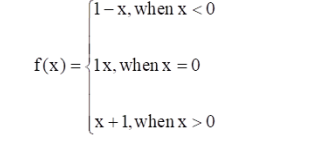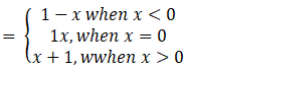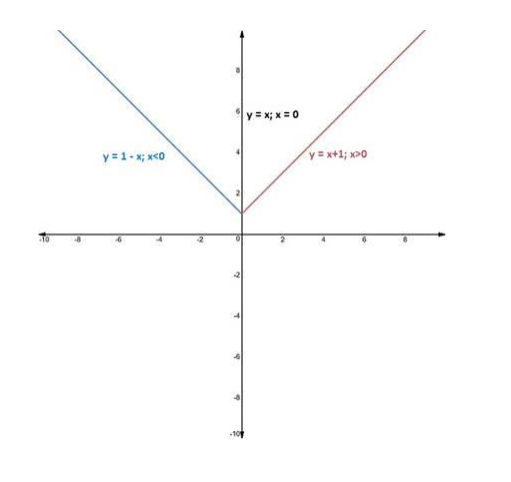# Consider the function

Question:

Consider the function $\mathrm{f}: \mathbf{R} \rightarrow \mathbf{R}$, defined byWrite its domain and range. Also, draw the graph of f(x).

Solution:

Given:

f(x)To Find:

Domain and Range of $\mathrm{f}(\mathrm{x})$

When $f(x)=1-x \mid x<0$

In this case there is no value of $x(x<0)$ which makes the above expression undefined.

Therefore

Domain(f) $=(-\infty, 0) \ldots(1)$

When f(x) = x | x = 0

In this case there is no value other than 0 which makes the above expression undefined.

Therefore

Domain(f) $=0 \ldots(2)$

When f(x) = x + 1 | x > 0

In this case there is no value of x (x > 0) which makes the above expression undefined.

Therefore,

Domain(f) $=(0, \infty) \ldots(3)$

From equations (1),(2) \& (3) We can say that the domain of $f(x)$ as a whole :

Domain $(f)=(-\infty, \infty)$

Now when, $f(x)=1-x$

$x=1-f(x)$

As $x$ ranges from $-\infty$ to 0 , then $f(x)$ ranges from 1 to $\infty$

Therefore,

Range $(f)=(1, \infty) \ldots(4)$

Now when, $f(x)=x$

As x = 0

Therefore,

Range(f) = 0 …(5)

Now when, f(x) = x +1

$x=f(x)-1$

As $x$ ranges from 0 to $\infty$, then $f(x)$ ranges from 1 to $\infty$

Therefore,

Range(f) $=(1, \infty) \ldots(6)$

From (4), (5) & (6) the range of f(x) as whole:

Range $(f)=0 \cup(1, \infty)$

Graph: